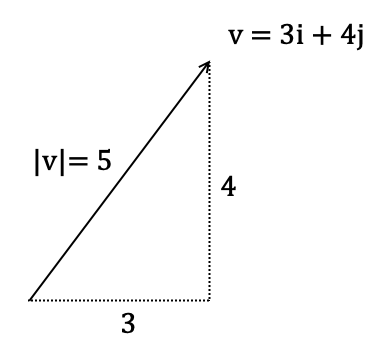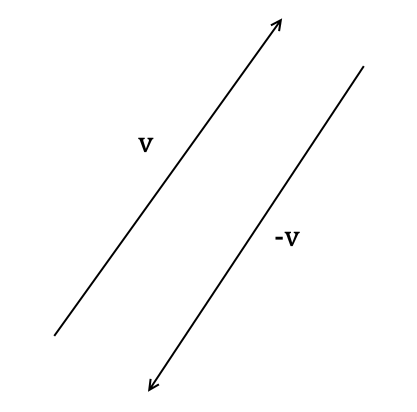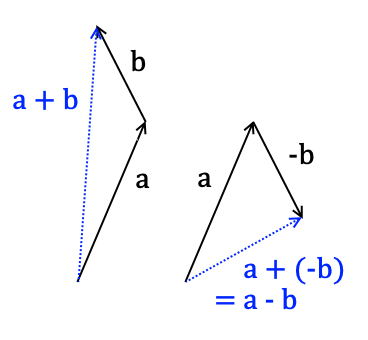Vectors and motion

Introduction

You are probably quite familiar with the concepts of distance and speed.

We extend these physical quantities by introducing vectors, which allow us to set a direction as well as a magnitude.

Here's a vector that I've called $\mathbf{v}$

$$\mathbf{v} = 3\mathbf{i}+4\mathbf{j}$$

Think of this vector as 'three along, four up'. $\mathbf{i}$ and $\mathbf{j}$ are themselves vectors, called unit vectors in the $x$ and $y$ directions respectively. Think of $\mathbf{i}$ as 'right' and $\mathbf{j}$ as 'up'.

The modulus of a vector $|\mathbf{v}|$ is its length. In mathematical terms, for some vector $\mathbf{v} = x\mathbf{i}+y\mathbf{j}$ its modulus is found using pythagoras

$$|\mathbf{v}| = \sqrt{x^{2}+y^{2}}$$

The modulus of a vector often describes some physical quantity in scalar form, just a number, instead of vector form. You will see why that is useful in a minute.

Most students find a visual example more explanatory. Here is what my previous vector $\mathbf{v}$ looks likeObserve that the vector has a defined length and direction. I have marked the horizontal and vertical components on the diagram.

The direction and magnitude of a vector are entirely determined by its horizontal and vertical components, the coefficients of $\mathbf{i}$ and $\mathbf{j}$.

You can multiply a vector by a scalar quantity to change its magnitude.

$$k\mathbf{v} = kx\mathbf{i}+ky\mathbf{j}$$

For $k\gt 0$ the direction of the vector will remain constant, but $k\lt 0$ will make the vector go in the opposite direction. Indeed $-\mathbf{v}$ has the opposite direction to $\mathbf{v}$.Vector addition is achieved by adding together the horizontal components of both vectors together and the vertical components together. The same goes for subtraction. The result of this is called the resultant vector.

Vector addition can be visualised by placing one vector at the end of another. For subtraction we do something similar.Bearings

A vector's bearing is the angle in degrees from the vertical north line, written as three digits from 000° and 180°. A bearing of 060° would indicate the vector points in the direction 60° from the north line.

Displacement, velocity, and acceleration

I will now define several vector quantities related to motion that you need to learn.

Displacement is a vector quantity that describes how far an object has moved from a set original position, and in which direction. It is measured in metres, abbreviated $\textrm{m}$.

Displacement is denoted $\mathbf{s}$, deriving from the Latin 'spatium'. It is best thought of as an object's 'relative position' in space. The modulus of a displacement vector is distance, also measured in metres.

Velocity is another vector quantity that describes how quickly an object is moving and in which direction it is moving in. It is measured in metres per second, abbreviated $\textrm{ms}^{-1}$.

We sometimes distinguish between an object's initial velocity and final velocity over a given time period. These are denoted $\mathbf{u}$ and $\mathbf{v}$ respectively. The modulus of a velocity vector is speed, also measured in $\textrm{ms}^{-1}$.

We also use the term average velocity, which is the arithmetic mean of the initial and final velocities

$$\frac{\mathbf{u} + \mathbf{v}}{2}$$

Now to link all of these quantities together. Just as we define the scalar quantities

$$\textrm{speed} = \frac{\textrm{distance}}{\textrm{time}}$$

We define the relationship between them in vector form

$$\mathbf{v} = \frac{\mathbf{s}}{t}$$

Where $\mathbf{v}$ is average velocity in $\textrm{ms}^{-1}$, $\mathbf{s}$ is displacement in $\textrm{m}$, and $t$ is time measured in seconds, abbreviated $\textrm{s}$.

Example

Q) After 5 seconds of motion a particle's displacement is found to be $\left(2\mathbf{i}+\mathbf{j}\right)$ metres. Find its average velocity during this motion.

A) $\mathbf{v} = \frac{\mathbf{s}}{t}$ so we have

$$\mathbf{v} = \frac{2\mathbf{i}+\mathbf{j}}{5} = \left(\frac{2}{5}\mathbf{i}+\frac{1}{5}\mathbf{j}\right) \textrm{ms}^{-1}$$

Example

Q) A car drives on a straight section of a racing track aligned due east, and is found to travel the 100m distance in 5 seconds. Find its average velocity during this motion.

A) $\mathbf{v} = \frac{\mathbf{s}}{t}$ so we have

$$\mathbf{v} = \frac{100\mathbf{i}}{5} = \left(20\mathbf{i}\right) \textrm{ms}^{-1}$$

Example

Q) A man walks with an average velocity of $\left(2\mathbf{i}+2\mathbf{j}\right) \textrm{ms}^{-1}$ for 10 minutes, and then changes direction so he is walking due east with an average speed of $2.5\textrm{ms}^{-1}$ for the next 15 minutes. What is his displacement from his initial position?

A) $\mathbf{v} = \frac{\mathbf{s}}{t}$ so rearranging gives $\mathbf{s}=\mathbf{v}t$. Therefore the displacement vector $\mathbf{s}_{1}$ after the first 10 minutes is

$$\mathbf{s}_{1} = \left(2\mathbf{i}+2\mathbf{j}\right)\times 600 = \left(1200\mathbf{i}+1200\mathbf{j}\right)\textrm{m}$$

In the second portion his velocity is $\left(2.5\mathbf{i}\right)\textrm{ms}^{-1}$. Therefore in that portion he travels

$$\mathbf{s}_{2} =\left(2.5\mathbf{i}\right)\times 900 = \left(2250\mathbf{i}\right)\textrm{m}$$

Adding together the two displacement vectors gives us the resultant displacement from the original point.

$$\mathbf{s}_{1} + \mathbf{s}_{2} = \left(1200\mathbf{i}+1200\mathbf{j}\right) + \left(2250\mathbf{i}\right) = \left(3450\mathbf{i}+1200\mathbf{j}\right)\textrm{m}$$

Note that it is not the total distance he walks along his line of travel. Displacement is the shortest straight line from his initial starting point to his ending point.

Changing velocity

In real life situations velocity doesn't always stay constant. Acceleration is the rate of change of velocity, a vector quantity denoted $\mathbf{a}$ and measured in metres per second per second, abbreviated $\textrm{ms}^{-2}$.

$$\textrm{acceleration} = \frac{\textrm{final velocity}-\textrm{initial velocity}}{\textrm{time}}$$

And in vector form

$$\mathbf{a} = \frac{\mathbf{v}-\mathbf{u}}{t}$$

Where $\mathbf{v}$ and $\mathbf{u}$ are the final and initial velocities respectively, both measured in $\textrm{ms}^{-1}$ and $t$ is time in $\textrm{s}$.

Example

Q) A dog sees a cat at the end of a garden due north, and starts running towards it, accelerating from rest up to a velocity of $\left(8\mathbf{j}\right)\textrm{ms}^{-1}$ in 3 seconds. Find his acceleration in this time.

A) The dog started from rest so his initial velocity is 0. His final velocity $\left(8\mathbf{j}\right)\textrm{ms}^{-1}$, and he makes this change in velocity in 3 seconds.

$$\mathbf{a} = \frac{\mathbf{v}-\mathbf{u}}{t} = \frac{8\mathbf{j}-0}{3} = \left(\frac{8}{3}\mathbf{j}\right)\textrm{ms}^{-2}$$

Force

Intuitively you will know that applying a force to something makes it move.

Mathematically we can model force as a vector $\mathbf{F}$ just as we can the other physical quantities like velocity and acceleration. Force is measured in units called Newtons, abbreviated $\textrm{N}$.

And just like the other physical vector quantities, you can add different forces together on the same object to get a resultant force.

Example

Q) A particle of mass 5kg is pushed with two forces, the first one $\mathbf{F}_{1} = \left(-\mathbf{i}+\mathbf{j}\right)\textrm{N}$ and the second one $\mathbf{F}_{1} = \left(\mathbf{i}+3\mathbf{j}\right)\textrm{N}$. Is the object in equilibrium? If not, calculate its acceleration due to the resultant force.

A) We have $m=5\textrm{kg}$, and the resultant of the two forces is their sum

\begin{align} \sum \mathbf{F} &= \mathbf{F}_{1} + \mathbf{F}_{2} \\ &= \left(-\mathbf{i}+\mathbf{j}\right) + \left(\mathbf{i}+3\mathbf{j}\right) \\ &= \left(4\mathbf{j}\right)\textrm{N} \end{align}

The resultant force is non-zero so the object is not in equilibrium. Use N2L to find its acceleration

$$\sum \mathbf{F} = m\mathbf{a} \Rightarrow \mathbf{a} = \frac{1}{m} \sum \mathbf{F} = \frac{1}{5}\left(4\mathbf{j}\right) = \left(\frac{4}{5}\mathbf{j}\right)\textrm{ms}^{-2}$$

Position vector

An object's position vector describes its location in two dimensional space at some time. It is a vector function of time $t$ denoted $\mathbf{r}\left(t\right)$ and measured in metres.

An intuitive way to think of it is

$$\mathbf{r}\left(t\right) = \left( \textrm{original starting position} \right) + \left( \textrm{velocity} \right)\times t$$

To form a position vector for an object you don't need to know $t$ to begin with. It is simply a function that tells you where an object will be at some time, given an initial starting point and a velocity vector.

Example

Q) A hiker starts walking from a point 1m due east of a style across a field with velocity $\left(\mathbf{i}-3\mathbf{j}\right)\textrm{ms}^{-1}$. Find his position vector at time $t$ relative to the style.

A) His initial starting position was $\left(\mathbf{i}\right)\textrm{m}$ relative to the style and his velocity is given as $\left(\mathbf{i}-3\mathbf{j}\right)\textrm{ms}^{-1}$. Therefore his position vector at time $t$ is

\begin{align} \mathbf{r}\left(t\right) &= \mathbf{i} + \left(\mathbf{i}-3\mathbf{j}\right)t \\ &= \left(1+t\right)\mathbf{i} -\left(3t\right)\mathbf{j} \end{align}

We can also use the position vectors of two objects to find if they meet, and if so at what time they meet.

Two objects will meet in two dimensional space at time $t_{m}$ if and only if

$$\mathbf{r}_{1}\left(t_{m}\right) = \mathbf{r}_{2}\left(t_{m}\right)$$

In practical terms we can work out $t_{m}$ by taking

$$\mathbf{r}_{1}\left(t_{m}\right) - \mathbf{r}_{2}\left(t_{m}\right) = 0$$

And solving for $t_{m}$.

Example

Q) In the same situation as the example above, a dog is 2m due south of the hiker at time $t=2\textrm{s}$. At that instant, the dog starts running so that they meet at $t=8\textrm{s}$. If the hiker's position vector is the same as before, find the position vector of the dog at time $t \ge 2$.

A) We'll label the position vectors of the hiker and the dog $\mathbf{r}_1 \left(t\right)$ and $\mathbf{r}_2 \left(t\right)$ respectively. In the last question we found that

$$\mathbf{r}_1 \left(t\right) = \left(1+t\right)\mathbf{i} -\left(3t\right)\mathbf{j}$$

And we're told that the dog is 2m due south of the hiker at time $t=2\textrm{s}$. The position of the hiker at that time is

$$\mathbf{r}_1\left(2\right) = 3\mathbf{i}-6\mathbf{j}$$

Therefore the dog's position vector at that time is

$$\mathbf{r}_2\left(2\right) = 3\mathbf{i}-8\mathbf{j}$$

We now have the dog's starting position. We're then told that the hiker and the dog meet at $t=8\textrm{s}$. If we let the dog's velocity be $\left(a\mathbf{i}+b\mathbf{j}\right)\textrm{ms}^{-1}$, then we can say

\begin{align} \mathbf{r}_1 \left(8\right) &= \mathbf{r}_2 \left(8\right) \\ \left(1+8\right)\mathbf{i} -\left(3\times 8\right)\mathbf{j} &= 3\mathbf{i}-8\mathbf{j} + \left(a\mathbf{i}+b\mathbf{j}\right)\times 8 \\ 9\mathbf{i} -24\mathbf{j} &= \left(3+8a\right)\mathbf{i}+\left(8b-8\right)\mathbf{j} \end{align}

Comparing coefficients of $\mathbf{i}$ and $\mathbf{j}$ we can form two equations

\begin{align} 3 + 8a &= 9 \\ 8b - 8 &= -24 \end{align}

These can easily be solved to find $a = \frac{3}{4}$ and $b=-2$. Therefore the dog's position vector at time $t \ge 2$ is

$$\mathbf{r}_2 \left(t\right) = \left(3 + \frac{3}{4}t\right)\mathbf{i} - \left(8+2t\right)\mathbf{j}$$